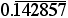# Creating Integers

Find a positive integer so that when you multiply 0.20 by that integer, the product is an integer. What is the smallest positive integer that will “work”? What are the smallest positive integers that will work for other decimals such as 0.05, 1.25, 0.375, 0.444…,Compare your answers with the fraction equivalents of 0.20, 0.05, 1.25, 0.375, 0.444,… andDescribe how a fraction equivalent can be determined from a decimal number.Extension
Will this method work for all decimal numbers?